# Recursion in C#

## Recursion in C# with Examples

In this article, I am going to discuss Recursion in C# with Examples. Please read our previous article where we discussed Call by Value and Call by Reference in C# Language with Examples. At the end of this article, you will understand the following pointers in detail.

1. What is Recursion in C#?
2. What does it mean by Recursive Function in C#?
3. How does Recursion Work in C#?
4. How to trace a Recursive Function in C#?
5. Example to Understand Recursion in C#
6. What are the advantages of Recursion in C# Language?
7. What are the disadvantages of Recursion in C# Language?
8. How to Find the Time Complexity of a Recursive Function in C#?
##### What is Recursion in C#?

Before understanding Recursion, first, let us have a look at the below code. Here we have two functions i.e. the Main function and the fun function and the fun function is called by the Main function.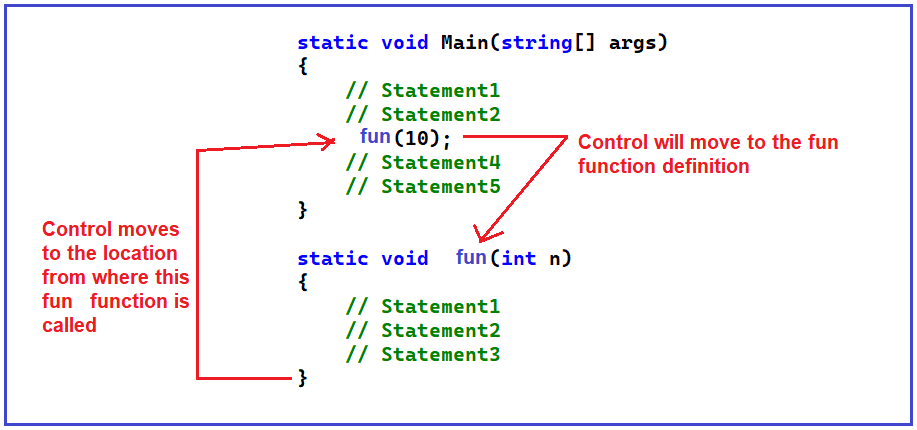First, we need to understand how this function call is made and how it works. Here, once the program execution starts, it will start executing the program from the Main method. First, it will execute the first statement, then it will execute the second statement and then it will execute the third statement i.e. it will call the fun function. Here, the control will move to the fun function definition and start executing that fun function. Inside the fun function, it will start executing the first statement, then the second, and then the third statement. Once it has finished (once the third statement is executed inside the fun function) the control again comes back to the same line i.e. third line of the Main function from where the fun function is called. If any other operations are present in that line they will execute. Otherwise, it will execute the fourth statement and then the fifth statement, and so on.

##### What does it mean by any other operations?

Let’s say the fun function is returning something and in the Main function, we have written added by 2 with the fun function call i.e. fun(1) + 2. So, the returning value from the fun function has to be added by two. And this addition needs to be done once the fun function is returned to the Main function with some value. Assume that the fun function has a return value of 100. So, 100+2 can be done only if the fun(10) has returned the value. This is the important point that you should remember for understanding the recursion. For a better understanding, please have a look at the below image.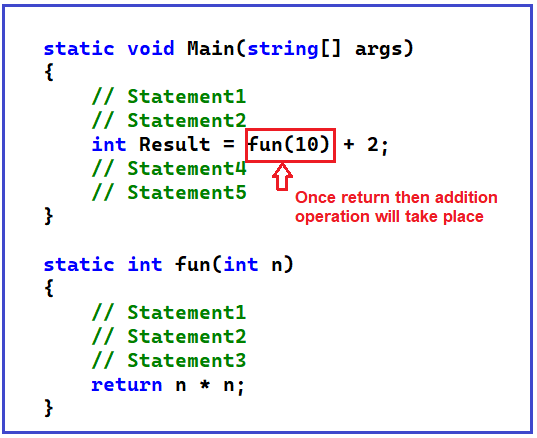To understand the recursive function, we need to understand the working flow of the following example. In the below example, the program execution is started from the Main method. From the Main method, the function Fun1 is called, and from the Fun1 function Fun2 method is called. Again, from the function Fun2, the Fun3 method is called, and finally, from function Fun3, the function Fun4 is called.

```using System;
namespace RecursionDemo
{
class Program
{
static void Main(string[] args)
{
Console.WriteLine("Main Method Started");
fun1(4);
Console.WriteLine("Main Method Started");
}
static void fun1(int n)
{
Console.WriteLine("Fun1 Started");
fun2(3);
Console.WriteLine("Fun1 Ended");
}
static void fun2(int n)
{
Console.WriteLine("Fun2 Started");
fun3(2);
Console.WriteLine("Fun2 Ended");
}

static void fun3(int n)
{
Console.WriteLine("Fun3 Started");
fun4(1);
Console.WriteLine("Fun3 Ended");
}

static void fun4(int n)
{
Console.WriteLine("Fun4 Started");
Console.WriteLine("Fun4 Ended");
}
}
}
```
###### Output: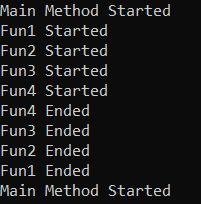The point that we need to understand is when the Main method, Fun1, Fun2, Fun3, and Fun4 method execution is going to be completed. As you can see in the above output, first the Main method, then the Fun1 method, then Fun2, then Fun3, and then the Fun4 method execution started. But, first Fun4 method execution ended, then the Fun3 execution ended, then Fun2, then Fun1, and finally Main method execution ended.

The point that you need to remember is that when we called a method (let’s say F2) from another method (let’s say F1), then the F1 method execution is only going to be completed, once the F2 method execution is completed. That means first the called method execution needs to be completed and then only the caller method execution going to be completed. But this is not the case in asynchronous programming. This is the case for synchronous programming. We will discuss Asynchronous Programming in our upcoming articles. With this kept in mind, let us proceed and understand what is a Recursive Function in C#.

##### What does it mean by Recursive Function in C#?

Function calling itself is called Recursion. Or in simple words we can say that recursion is a process in which a function calls itself repeatedly until some specified condition has been satisfied. It is similar to a loop, in the loop, as long as the loop condition is satisfied, the loop executes and in the same manner, as long as the condition is satisfied, the function will call itself.

In order to solve a problem recursively, two conditions must be satisfied. First, the problem must be written in a Recursive Form so that the function will call itself, and second, the problem statement must include a Stopping Condition so that we can stop the function call.

The most important point that you need to remember is that if a recursive function contains any local variables, a different set of local variables will be created during each call. The variables will represent a different set of values each time the function is executed. Each set of values will be stored on the stack memory. If this is not clear at the moment, then don’t worry, we explain these things when we start discussing the examples. The general form of recursion is given below.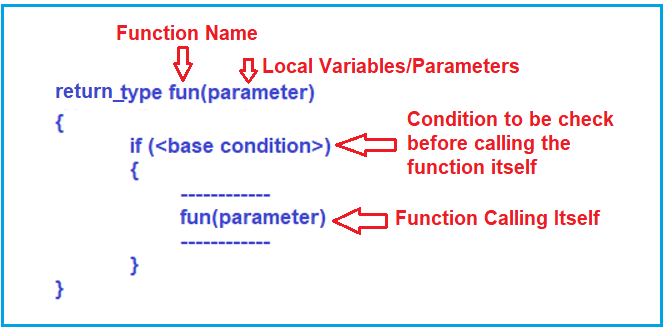This is the general form of a recursive function i.e. a function is calling itself. Inside the function body, if you see if it is calling itself again and again, then it is a recursive function.

One more important point that you need to remember is that inside a recursive function, you can see that there is a base condition. That means there must be some base condition to terminate the recursion. It is similar to a loop, if you are having a loop, and if there is no condition to terminate the loop, then you will have an infinite loop. So, there must be some ways to terminate the recursion otherwise it will go into infinite calling. First, we need to call the function for the first time, then from the second time onwards, it will call itself repeatedly again and again. So, there must be some condition under which it must stop.

As you can see in the above image, the function will call itself as long as the base condition is true. Here if the condition becomes false it will not call further and it stops. This is how recursion works in C# language. Now, let us proceed further and see some examples to understand the recursion and how exactly the recursion works.

##### How does Recursion Work in C#?

Let us look at an example to understand how recursion works. Please have a look at the following example. Here, we have the Main function which is having some value in the variable x, and then it is calling the fun1 function passing that variable X value. The function fun1 that takes parameter n will accept the x value and if the condition is ‘true, it is printing the value and then calling itself. So, here, it is printing and again calling itself for a reduced value of n.

```using System;
namespace RecursionDemo
{
class Program
{
static void Main(string[] args)
{
int x = 3;
fun1(x);
}

static void fun1(int n)
{
if (n > 0)
{
Console.Write(\$"{n} ");
fun1(n - 1);
}
}
}
}
```

In the above example, we are passing 3 to the fun1 function from the main function. Let us see what will be the result and how it works. Let’s, trace this recursive function and check.

##### How to trace a Recursive Function in C#?

A recursive function is traced in the form of a tree. So, let us start tracing the above example. When the condition is true inside the fun1 function there are two statements to be executed. In the first statement, it will print the n value and in the second statement it will call itself passing (n-1) and this has to be done only when n is greater than 0.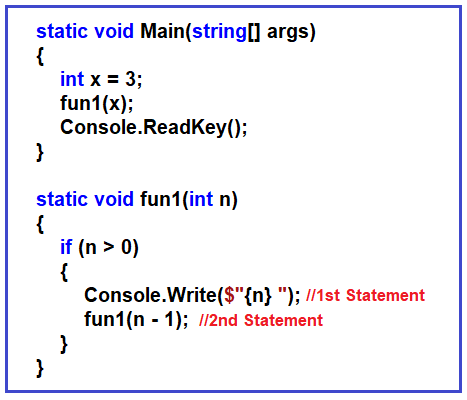###### fun1(3):

Let’s start tracing, from the main function, we are calling function fun1 passing X i.e. value 3. So, the first time the variable n has got the value 3, 3 is greater than 0 and hence the condition becomes true. So, the first step is to print n i.e. it will print 3 and the second step is to call itself again fun1 for 3-1 i.e. 2. Here, the fun1(3) call has not been completed. It calling itself again.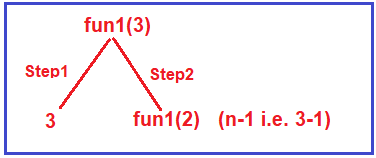###### fun1(2):

So, it will call itself again passing the n value as 2 i.e. fun1(2). So, let us execute fun1(2), again it will start and check the condition, now for this function call n value is 2, and 2 is greater than 0 and hence the condition becomes true. So, the first step is to print the n value i.e. it will print 2, and then call itself again by reducing the n value by 1 i.e. fun1(n-1) and the current n value is 2, so, it will call the function fun1(1). But remember, the fun1(2) call has not yet finished, it has only printed 2 and it has to call fun1(1).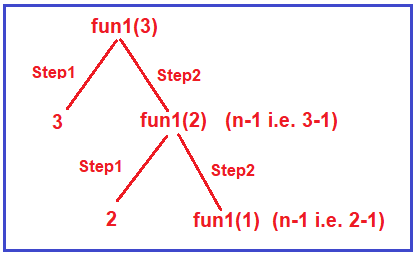###### fun(1):

So again, a new call, a fresh call, that fresh call is fun1(1). 1 is greater than 0, so we have to perform the two steps. The first step is to print 1 and then call itself by reducing the n value by 1 i.e. fun1(n-1) and the current n value is 1 so it will call fun1(0). But the point that you need to remember is that the fun1(1) call has not yet finished, it has printed 1 and it has to call fun1(0).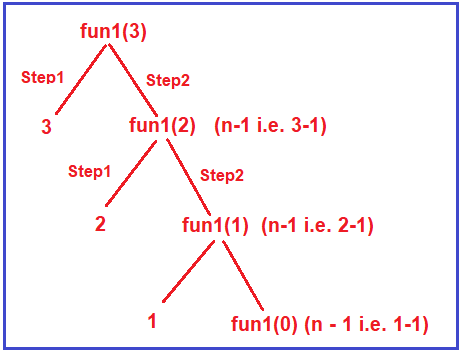###### fun1(0):

Now, fun1(0), i.e. the current n value for this call is 0 and it will check the condition i.e. 0 is greater than 0, and this time the condition becomes false. So, it will not enter inside the if block, it will not execute those two steps. So, this time there is no printing and no call, and after the if block, are there any statements to be executed? No, there are no statements after the if block to be executed. So, it will just come outside of the function. And that’s will end the fun1(0) call and from here, the control will go back to the previous function call and so on and finally come out from the fun1 to the main function where it is initially called. So, a recursive function forms a tree and this is called the tracing tree of a recursive function.

So, when you execute the above example, you will get the output as 3 2 1. Now we will take one more example.

##### Example to Understand Recursion in C#:

Let us understand Recursion in C# with another example. Please have a look at the below example which is also an example of the recursive function in C#.

```using System;
namespace RecursionDemo
{
class Program
{
static void Main(string[] args)
{
int x = 3;
fun2(x);
}

static void fun2(int n)
{
if (n > 0)
{
fun2(n - 1);
Console.Write(\$"{n} ");
}
}
}
}
```

The above example is very similar to the first example that we have just discussed. Let me compare both examples and show you the difference.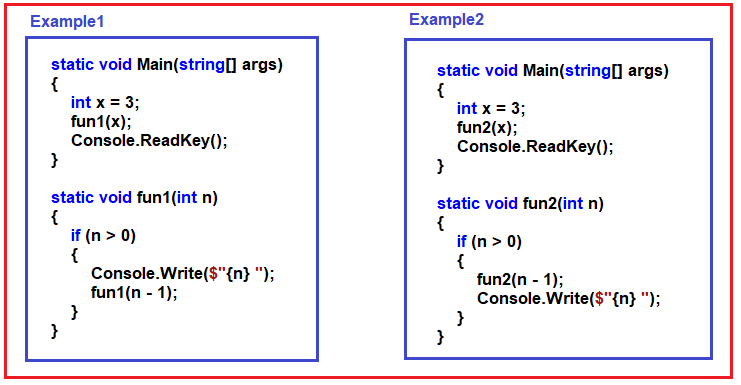If you look at the main function of both the example, they are having one variable called x and call one function (Example1 calling fun1 function and Example2 calling fun2 function) passing that x value.

The difference in both the example is that in example 1, inside the fun1 function if the condition is true (i.e. n > 0), first it is printing the n value and then calls itself but in example 2, inside the fun2 function if the condition is true (i.e. n > 0), first it is calling itself, and then printing the n value, then what will be the output. Let’s trace example 2 and find out the output.

###### fun2(3):

The program execution will start from the Main function. The Main function calls the function fun2 by passing the value 3 i.e. fun2(3). Inside the fun2 function, first, it will check whether n > 0 and here, n is 3, so 3 is greater than 0 and the condition is satisfied. So, the first statement within the if block is going to be executed i.e. it will call the fun2 function by passing n-1 i.e. 2. What about the second statement i.e. printing? It will not be executed at this point in time. The point that you need to remember is first the first statement has to be finished in order to execute the second statement i.e. printing. For a better understanding please have a look at the below image.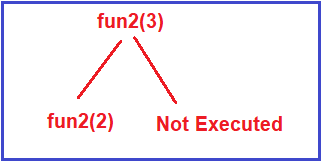###### fun2(2):

Let us take the fun2(2) call, with n=2, the condition again satisfied as 2 is greater than 0. Again, two steps, first it will call fun2 with n-1 i.e. it will call itself for n value of 1 i.e. fun2(1) and the second statement will not be executed at this point. Once the first statement execution is completed, then only the second statement going to be executed. At this point, the tracing tree will be like below.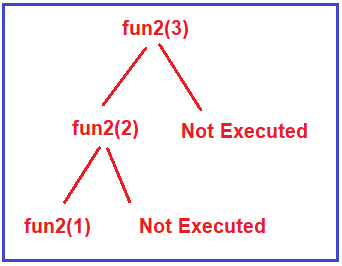###### fun2(1):

Let us trace fun2(1). Again 1 is greater than 0 and hence the condition is satisfied and again two steps. In the first step, it will call itself bypassing n-1 i.e. fun2(0), and similarly, the second statement going to be executed only once the first statement completes its execution. So, at this point, the tracing tree of this recursive function is like the one below.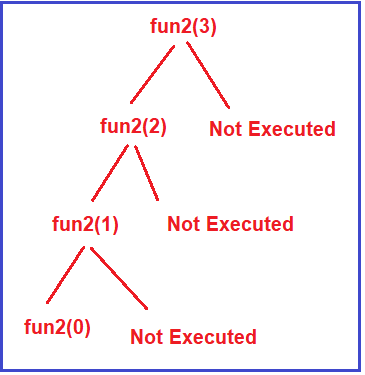###### fun2(0):

The next call is fun2(0). Now fun2(0), 0 is greater than 0, no. The condition is not satisfied. So, it will not enter inside this if block and it will come out i.e. it does nothing. So, this call with parameter 0 has terminated.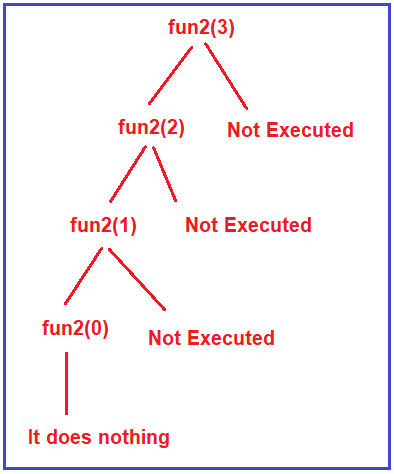Now once this call has been terminated the control will go back to the previous call. The previous call was fun2(1), it will go back to the function call and execute the next statement i.e. the second statement which is nothing but to print the n value. At this call, the n value is 1 and hence it will print 1. For a better understanding, please have a look at the below image.Then it will go back to the previous call i.e. fun2(2), and the second thing that is remaining here is printing, so the value 2 is printed then it will come out of this function and finish. For a better understanding, please have a look at the following image.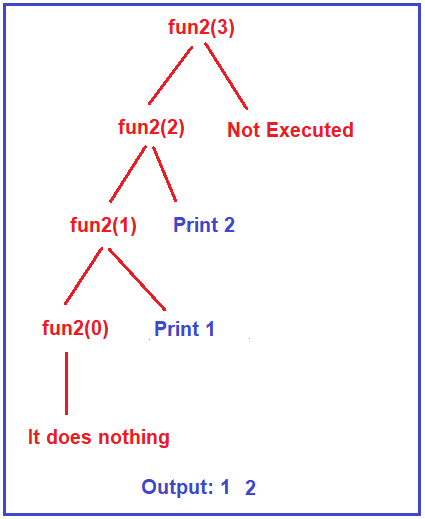Once the fun2(2) call finishes, it goes back to the previous call i.e. fun2(3), and the second thing that is remaining here is printing, so the value 3 is printed. And the output you will get from this function is 1 2 3 as shown in the below image.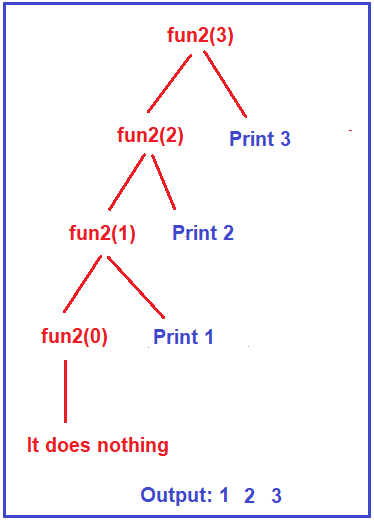Once you complete the fun(3) execution, the control will come back to the Main method, from where we call the fun1 function. So, the output of example 1 was 3, 2, 1 and the output of example 2 is 1, 2, 3.

Now, let us compare both of the examples, in example 1, first, the printing was done and then the recursive call was made but in example 2, first the recursive call was made and then the printing was done at returning time.

Note: The most important point that you need to understand in recursion is that recursion has two phases. One is the calling phase and the other one is returning phase.

##### Calculate the Factorial of a Number using Recursion:

In the below example, we declare our recursive factorial function which takes an integer parameter and returns the factorial of this parameter. This function will call itself with the decreased value of the number until the base condition is satisfied. When the condition is true, the previously generated values will be multiplied by each other, and the final factorial value is returned. We declare and initialize an integer variable with the value 5 and then print its factorial value by calling our factorial function.

```using System;
namespace RecursionDemo
{
class Program
{
static void Main(string[] args)
{
int x = 5;
Console.WriteLine(\$"The factorial of {x} is {factorial(x)}");
}

static int factorial(int number)
{
if (number == 1)
{
return (1); /* exiting condition */
}
else
{
return (number * factorial(number - 1));
}
}
}
}
```

Output: The factorial of 5 is 120

Let us understand the output with the Tracing Tree. The following tracing tree represents the calling time of the Recursive function. When we pass the n value as 1, it will not call the function itself, rather it returns 1 to its previous call and the same process will continue until it reaches the n value of 5.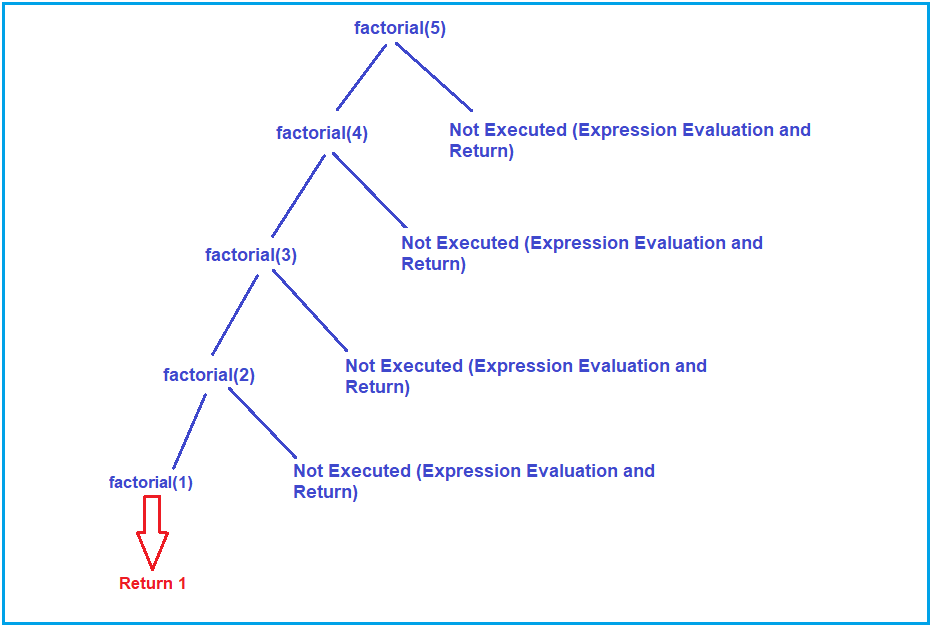The following tracing tree represents the returning time of the Recursive function.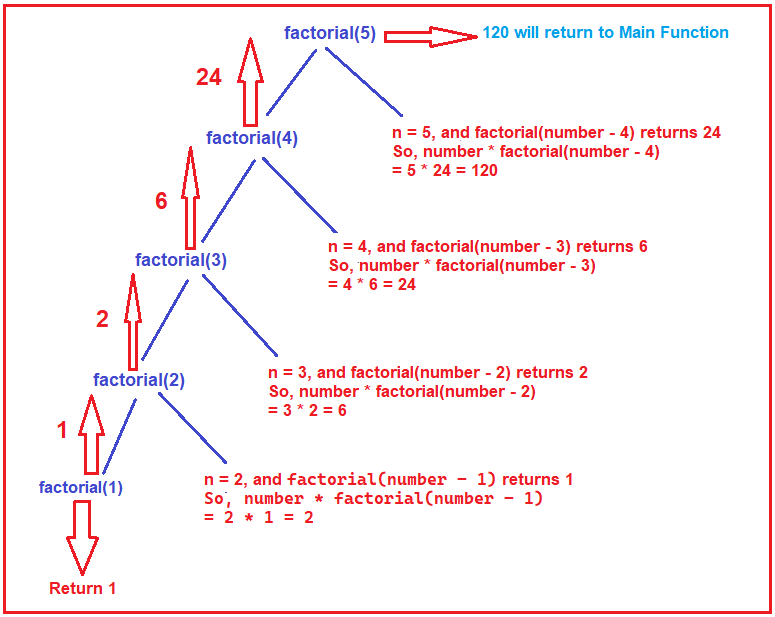##### What are the advantages of Recursion in C# Language?
1. Function calling-related information will be maintained by recursion.
2. Stack evaluation will take place by using recursion.
3. Prefix, postfix, and infix notation will be evaluated by using recursion
##### What are the disadvantages of Recursion in C# Language?
1. It is a very slow process due to stack overlapping.
2. The recursive program can create stack overflow.
3. The recursive program can create infinite loops.
##### How to Find the Time Complexity of a Recursive Function in C#?

Let us first understand the basic concept for finding the time complexity. We assume that every statement in our program takes one unit of time for execution.

Let me give the idea behind that one. Suppose, there are some books kept in one place and you have to move the book and keep it on a shelf or in a rack. How much time does it take? Maybe half a second, a quarter of a second, maybe if somebody works very slowly may take one second for keeping one book there. The time varies from person to person. So, we don’t mention seconds or milliseconds, we say one unit of time. If you take the example of currency, one dollar, one rupee, and one pound. We say one but what is the market value that might be different? So, we say one buck or one unit of currency.

In the same way, we assume that every statement takes one unit of time. If that statement is repeated multiple times, then we need to count the frequency that how many times it is executed. That is sufficient for analyzing our function.

##### Example to Find the Time Complexity of a Recursive Function in C#:

We are going to use the following recursive function to calculate the time complexity.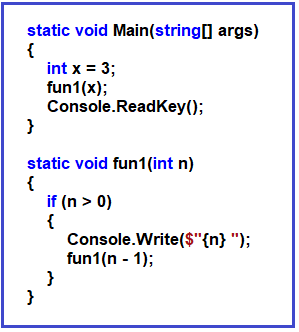Now, let us see what the above function (fun1) is doing. It is doing nothing just printing. It is just printing the value of n.

How much time does it take for printing? It takes one unit of time for printing.

How many times does the Console.Write() function is written there? Only one-time Console.Write() is written there. But this is a recursive function. So, it is calling itself again and again. As it is a recursive function, let us find out how many times the Console.Write() function is executed. As we already discussed, we can find out this using the tracing tree or the recursion tree.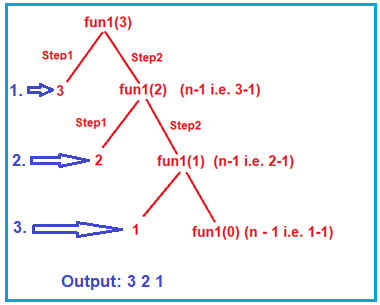As you can see in the above tracing tree, first it prints the value 3, then print 2 and then print the value 1. That means the Console.Write() statement is executed three times. So, this recursive function will take 3 units of time to execute when the n value is 3. If we make the n value 5 then it will take 5 units of time to execute this recursive function.

So, we can say for n it will take n units of time. Coming back to the example, if we have to keep one book on a shelf. You will take one unit of time, and for 10 books you will take 10 units of time. So, for n number of books, you will take n units of time. The most important point that you need to remember is time depends on the number of books. The time can be represented as the order of n i.e. O(n). The time taken is in order of n.

##### How do the variables work in a Recursive Function?

Let us see how variable works with a recursive function with an example. We have already discussed how to trace recursive functions. For a better understanding, please have a look at the following example.

```using System;
namespace RecursionDemo
{
class Program
{
static void Main(string[] args)
{
int number = 5;
int Result = fun(number);
Console.WriteLine(Result);
}
static int fun(int n)
{
if(n > 0)
{
return fun(n - 1) + n;
}
return 0;
}
}
}
```

As you can see in the above code, there is a function called fun which takes one parameter i.e. n of type integer. Then if the n value is greater than 0, it will call itself with a decreased value of n (i.e. n – 1) and also add n. So, when this plus n (i.e. +n) will be done (calling time or returning time)? It is done at returning time. If the value of n is 0, then it returns 0. From the main function, we called the fun function passing a i.e. 5. Let us trace the above recursive function. The following image shows the tracing of the fun call.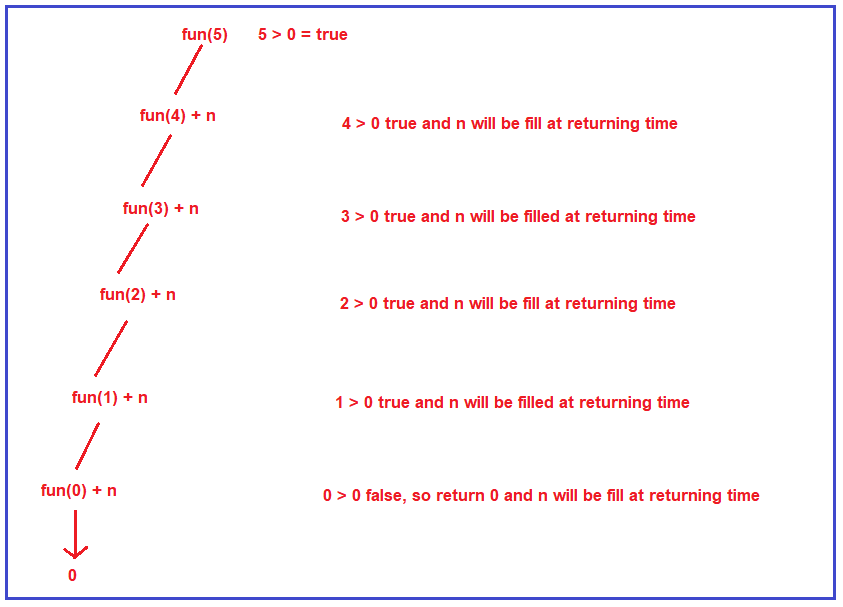First, the function fun is called for the value 5, and is 5 greater than 0? Yes, so it will call itself with the reduced value of n i.e. 4, and n i.e. 5 will be added at returning time. Then it will check whether 4 is greater than 0, yes, so it will call itself again with a reduced value of n i.e. 3 and the current n value i.e. 4 will be added at returning time. In this way, it will call itself until the n value becomes 0. When the n value becomes 0, the condition becomes false and it will not call itself rather it simply returns 0. From this onward the returning will happen and to the result of each function call, the n value will be added. For a better understanding, please have a look at the below image.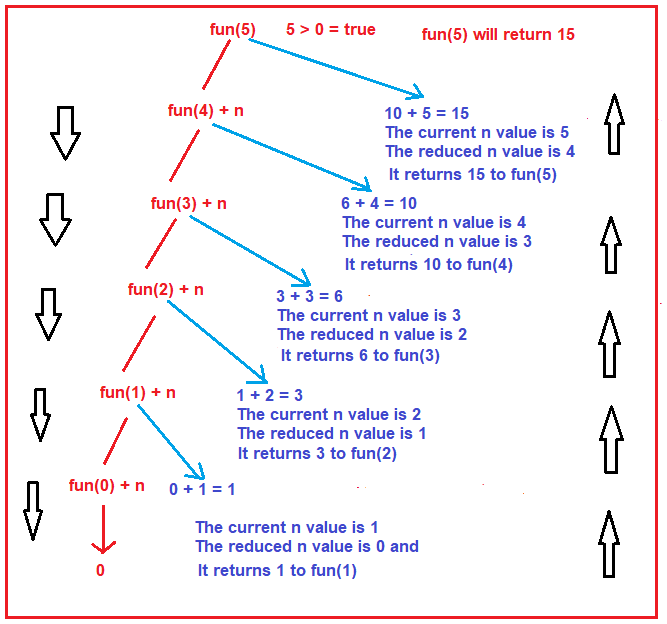Let us understand how the return will happen step by step

1. Fun(0) + n: In this case, the current n value is 1 and the reduced n value is 0, and fun(0) will return 0 and the current n value i.e. 1 will be added with the result of fun(0). So, this will return 1 to the previous function call i.e. fun(1) i.e. the result of the fun(1) function will be 1.
2. Fun(1) + n: In this case, the current n value is 2 and the reduced n value is 1, and fun(1) returns 1 (the output of the previous function call) and the current n value i.e. 2 will be added with the result of fun(1). So, this will return 3 to the previous function call i.e. fun(2) i.e. the result of the fun(2) function will be 3.
3. Fun(2) + n: In this case, the current n value is 3 and the reduced n value is 2, and fun(2) returns 3 (the output of the previous function call) and the current n value i.e. 3 will be added with the result of fun(2). So, this will return 6 to the previous function call i.e. fun(3) i.e. the result of the fun(3) function will be 6.
4. Fun(3) + n: In this case, the current n value is 4 and the reduced n value is 3, and fun(3) returns 6 (the output of the previous function call) and the current n value i.e. 4 will be added with the result of fun(3). So, this will return 10 to the previous function call i.e. fun(4) i.e. the result of the fun(4) function will be 10.
5. Fun(4) + n: In this case, the current n value is 5 and the reduced n value is 4, and fun(4) returns 10 (the output of the previous function call), and the current n value i.e. 5 will be added with the result of fun(4). So, this will return 15 to the previous function call i.e. fun(5) i.e. the result of the fun(5) function will be 15.

So, at the end fun(5) will return 15. This is the tracing of the above function when it is called with a value of 5. Now, let us see how the activation record is created. The activation record for the fun function will be created. For each value of n i.e. (5, 4, 3, 2, 1, 0) one activation record is created in the stack as shown in the below image. This is how the stack is created every time for each call.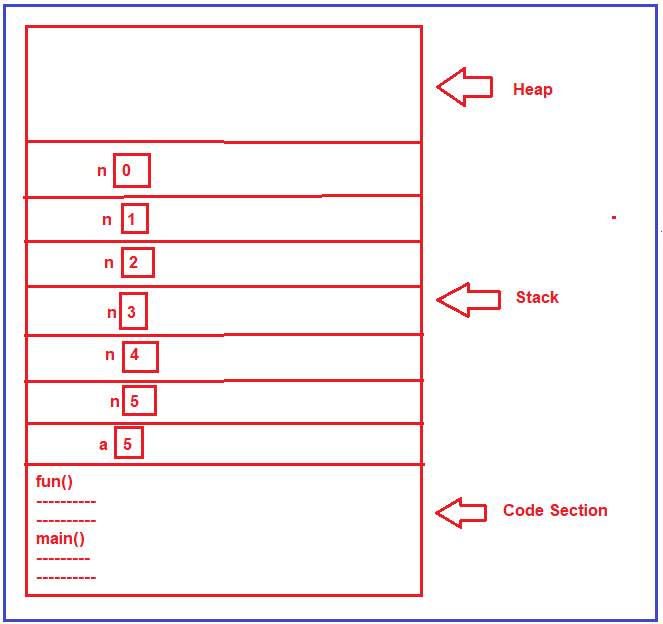In this case, you can see that the variable n is created 6 times in the stack area. We can write the above example using a loop that will only create the variable n only once. Let us rewrite the previous example using a loop.

```using System;
namespace RecursionDemo
{
class Program
{
static void Main(string[] args)
{
int number = 5;
int Result = fun(number);
Console.WriteLine(Result);
}
static int fun(int n)
{
int Result = 0;
for(int i = 1; i <= n; i++)
{
Result = Result + i;
}

return Result;
}
}
}
```

When you run the above example, you will get the same output as the previous example.

In the next article, I am going to discuss User Input and Output in C# with Examples. Here, in this article, I try to explain Recursion in C# with Examples. I hope you enjoy this Recursion in C# with Examples article. I would like to have your feedback. Please post your feedback, question, or comments about this Recursion in C# with Examples article.

### 2 thoughts on “Recursion in C#”

1.Thank you for such a detailed visual explanation

2.Best explanation for recursion on the internet (i read many article)!!!